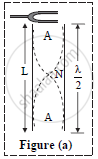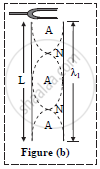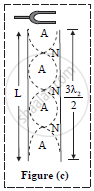# Discuss Different Modes of Vibrations in an Air Column of a Pipe Open at Both the Ends. - Physics

Discuss different modes of vibrations in an air column of a pipe open at both the ends.

Draw neat labelled diagrams for modes of vibration of an air column in a pipe when it is open at both ends

Hence derive an expression for fundamental frequency in each case.

#### Solution

First mode or fundamental mode:
In this mode of vibration, there is one node at the centre of the pipe and two antinodes, one at each open end as shown in figure (a).
Let,
v = wave velocity in air
n = fundamental frequency
λ = wavelength
L = length of air column
v = nλ ….(1)Also L=lambda/2

therefore lambda=2L ...................(2)

From Equation 1 and 2

v=n2L

n=v/(2L).......(3)

Equation (3) represents fundamental frequency or lowest frequency of vibration

ii. Second mode or first overtone:
In this mode of vibration, there are two nodes and three antinodes as shown in figure (b).Let,
v = wave velocity in air [As the medium is same, wave
velocity remains same]
n1 = next higher frequency
λ1 = corresponding wavelength
L = length of tube
Velocity of wave is given by,

v=n_1lambda_1    ..............(4)

Also L=lambda_1 ..............(5)

From equation (4) and (5),

v=n_1L

n_1=v/L

n_1=2xxv/(2L).................(6)

From equation (3) and (6)
 n_1 = 2n .…(7)

Thus, frequency of first overtone (second harmonic) is twice the fundamental frequency

iii. Third mode or second overtone:
In this mode of vibration, there are 3 nodes and 4 antinodes as shown in figure (C).
Let,
v = wave velocity in air (As the medium is same, wave velocity remains same)
n2 = next higher frequency
λ2 = corresponding wavelength
L = length of tube
Velocity of wave is given by,v=n_2lambda_2  ...................(8)

Also L=(3lambda_2)/2

lambda_2=(2L)/3

From equation (8) and (9),

v=n_2(2L)/3

n_2=(3v)/2L..............(10)

From equation (3) and (10),
n_2 = 3n

Thus, frequency of second overtone is thrice the fundamental frequency.

Concept: Study of Vibrations of Air Columns
Is there an error in this question or solution?
2012-2013 (October)

Share